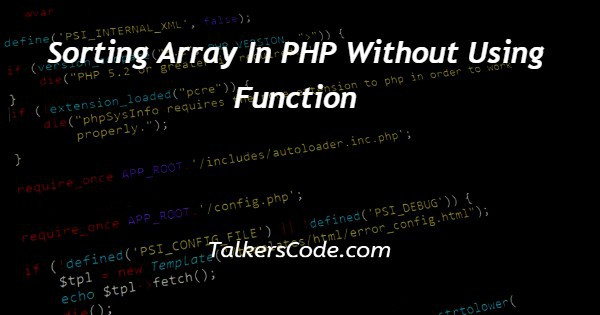# Sorting Array In PHP Without Using Function

Last Updated : Feb 2, 2023In this article we will show you the solution of sorting array in PHP without using function, here to achieve desired result we need to use two for loop to assess row and column wise.

First for loop refers first value then second loop points second onwards array values to compare with it.

Next if condition provide to compare remaining values one by one with the first value and sorting either ascending order or descending order.

This process repeated until last value of array to sort entire array values then printed at last.

## Step By Step Guide On Sorting Array In PHP Without Using Function :-

As foremost step, you should define array with more number within quotes symbol also give based on your wish.

Shown example array values can modifiable not mandatory and printed text by echo method with initial input array values.

Then first for loop defined with iterator variable ‘i’ within it defined another for loop with iterator ‘j’.

There to check condition of ascending comparison with remaining set of values in array and lastly we printed array once again to show sorted result of array.

To print array you should use print_r() or var_dump() methods both helps you to print array values.

```<?php
\$arr=array(
'12','34','4','9','8'
);
echo "Before Sort<br>";
print_r(\$arr);
for(\$i=0;\$i<count(\$arr);\$i++){
for(\$j=0;\$j<count(\$arr)-1;\$j++){
if(\$arr[\$j]>\$arr[\$j+1]){
\$t=\$arr[\$j+1];
\$arr[\$j+1]=\$arr[\$j];
\$arr[\$j]=\$t;
}
}
}
echo "<br>Sorted Array<br>";
print_r(\$arr);
?>```
1. A php script can be placed anywhere in the document. A php script starts with <?php and end with ?>.
2. The default file extension for php files is “.php” and php statements end with ‘;’ semicolon.
3. We defined array variable ‘\$arr’ with values ‘'12','34','4','9','8'’ and same as earlier said change this if you want, this is normal array.
4. Using echo we printed ‘Before Sort’ text at output with array value by print_r() method. To give clarification before and after sort array how to look.
5. First for defined with variable ‘i’ this array continues process until reach the end of length of array value. It is calculated by count() method then second loop defined inside first loop to point out next values to the first loop variable.
6. Here we used iterator ‘j’, which repeat the loop until last count. If condition defined that checks first value with next set of values if returns true then we replacing first value with next value by index.
7. This process repeated until thorough array sorted and then using print_r() we displayed array on the output it may vary if you changed array values otherwise you obtain result shown example with ascending order sort.

## Conclusion :-

In conclusion, now we are able to do sort without inbuilt function support in php.

When work with php we need to create and process php files at server location and then we need to start the server before execute the program.

When we executing this program on browser it will print ‘Before Sort Array (  => 12  => 34  => 4  => 9  => 8 ), Sorted Array

Array (  => 4  => 8  => 9  => 12  => 34 )’ at webpage browser.

I hope this article on sorting array in PHP without using function helps you and the steps and method mentioned above are easy to follow and implement.